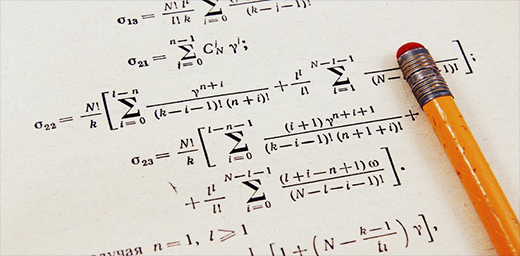Our Address: FZ LLC G 37 & G 34, Block 13, Al Sufouh Road Knowledge Park, Dubai PO Box : 501703

# THE SAT MATH: AN OVERVIEWThis article is intended to explore various areas of the SAT Math component, which is designed to align with the math you are learning in school. Working hard in your math class and applying those math skills to your science classes will give you the foundation you need for the SAT Math Test.

The raw score, which is the number of correct answers, on the test is converted into a scaled score between 200 and 800 for each section. As there is no negative marking on the test, skipped or wrong questions do not add or subtract from your raw score.

The Math Test has two portions. In the first section, you are given 55 minutes to complete 38 questions wherein calculators are permitted. This section of the test includes questions that involve more multifaceted mathematical approach. So, using a calculator would give you a chance to work more efficiently. Some problems, however, might be easier to solve without a calculator. So, it will be up to you to decide whether or not to use one.

The subsequent portion of the Math Test contains 20 questions, and 25 minutes will be allotted to complete it. Here, the test of your fluency with individual topics and concepts is the focus. Calculators are not permitted on this section, which adds a challenge to the exam. Multiple-choice forms the major chunk of the examination – around 80 percentage – while the other 20% are gridded response, which can include non-negative integers, fractions, or decimals. So, if you get a negative answer it is always a good idea to recheck your work. A set of reference formula will be provided at the beginning of the test. You may find these facts and formulas helpful as you answer some of the questions, but depending on them alone without having sufficient practice would not bring out good results as it slows you down. To do well, you should make sure you are already comfortable using them.

The Math test emphases on three main areas: Questions from the area we call “Heart of Algebra” necessitate you to create, manipulate, and solve algebraic equations. The next area is Problem Solving and Data Analysis, which examines your ability to use percentages, proportions, and ratios appropriately to solve problems in real-world situations, as well as to construe logic out from graphs and tables. The third area, which is the Passport to Advanced Math, involves questions that need you to demonstrate an acquaintance with more complex equations or functions. These are math skills you will want to master if you want to pursue a career in science, technology, engineering, or math.

On the Math test, there are a small number of questions that fall outside of the three main areas. These questions, collectively classified as ‘Additional Topics in Math’, will focus on certain key concepts, including area and volume, coordinate geometry, and basic trigonometry.

During the Math Test, some of the questions will be included from science and social science frameworks, in addition to other realistic settings.

You are in the right place to learn more about the SAT Math Test – right here on Option Training Institute. Time for some hands-on practice and real time ‘smart’ training. Let’s begin!